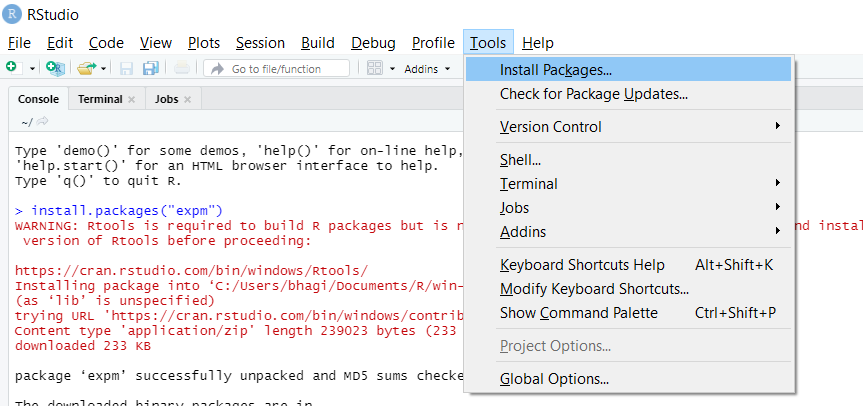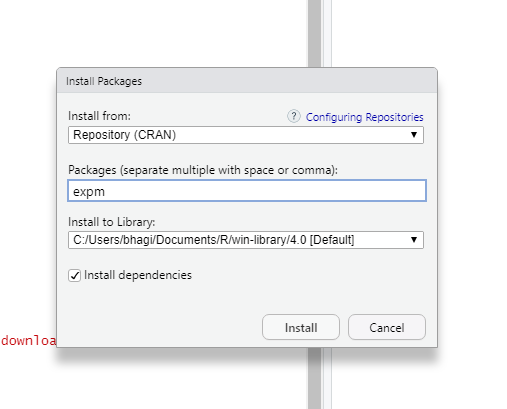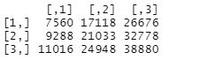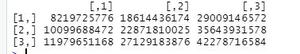Open in App
Not now

# Find the power of a matrix in R

• Last Updated : 05 Apr, 2021

In this article, we are going to see how to compute the power of a matrix in R Programming Language. Matrix is an arrangement of numbers into rows and columns.

Different ways of finding the power of matrix in R programming:

• By using %^%.
• By using a power function.

Method 1: By using %^%

Before using this we need to import expm library into our R studio.

Installing expm library into R studio:

Step 1: First you need to select tools.Step 2: After selecting the tool you need to press install packages:Below is the implementation:

We Import the expm and assigned values into the mat by using the matrix function. After that, we are finding the power of the matrix.

## R

 `# loading expm library``library``(expm) `` ` `# creating mat variable and storing ``# matrix into it.``mat <- ``matrix``(1:9, nrow=3)`` ` `# In matrix function data will fill column wise,``# here data will be 1 to 9 as we mentioned 1:9``# and rows will be 3 as we given nrows as 3`` ` `# finding power of matrix here power is 4``mat %^% 4`

Output:Method 2: By using a power function.

For using the power function we need to install matrixcalc package into our Rstudio

Below is the implementation:

Here we import the matrixcalc and assigned values into the mat by using matrix function. After that, we are finding the power of matrix by using power function.

## R

 `# loading package``require``(matrixcalc)`` ` `# creating matrix.``a<- ``matrix``(1 : 9, nrow = 3)`` ` `# finding power of matrix by using power function.``# In matrix function data will fill column wise,``# here data will be 1 to 9 as we mentioned 1:9``# and rows will be 3 as we given nrows as 3``matrix.power``(a, 9)`

Output:My Personal Notes arrow_drop_up